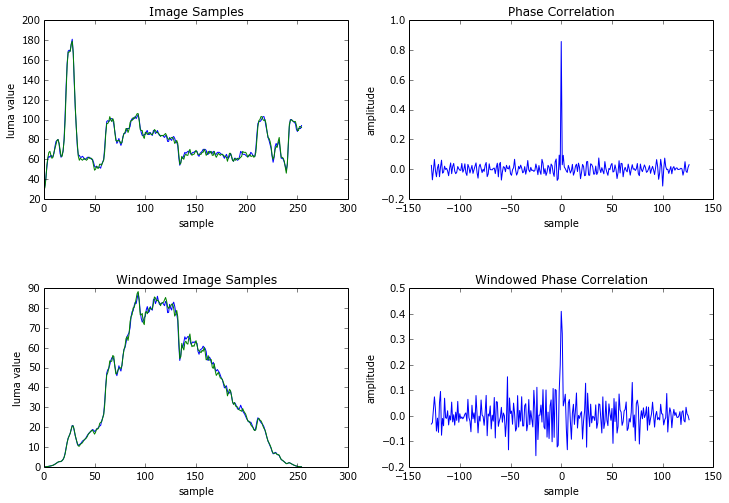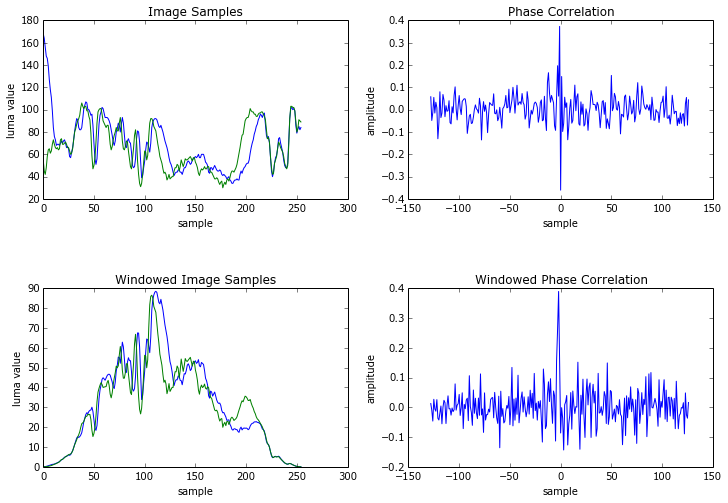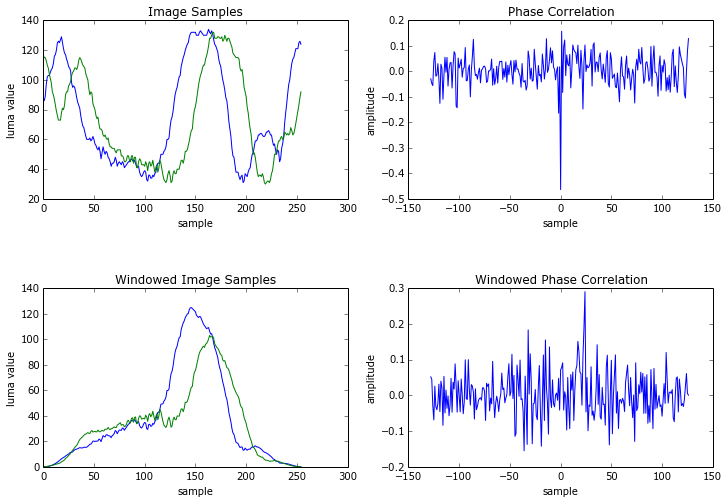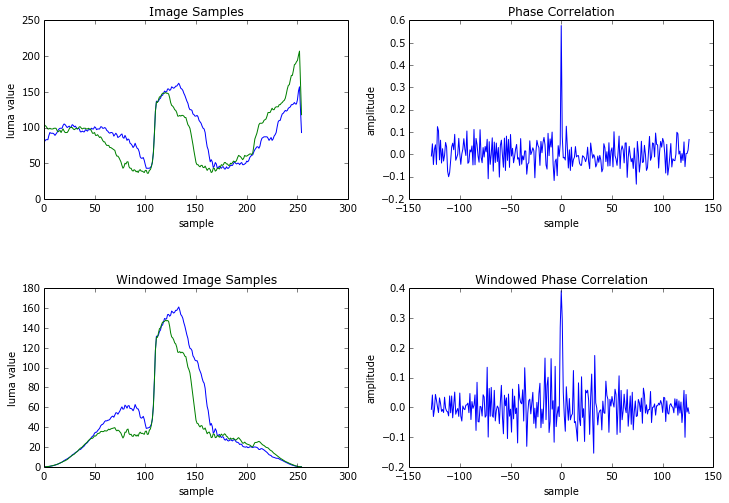# Phase Correlation for Motion Estimation - Part 1¶

### Overview¶

Phase correlation is a technique that can be used for motion estimation in various image processing applications such as video compression, but its use is uncommon. Spatial domain block matching techniques are much more commonly used.

### Purpose¶

Translate the mathematical equations for the phase correlation for images into practical code, and gain an conceptual understanding.

There are many references in the literature on Phase Correlation for motion estimation. I found these to be readily accessible.

Loy Hui Chien and Takafumi Aoki, "Robust Motion Estimation for Video Sequences Based on Phase-Only Correlation," Proceedings of the 6th IASTED International Conference, August 23-25, 2004, Honolulu, Hawaii, USA, pp. 1-6.

Yi Liang, "Phase-Correlation Motion Estimation," Digital Video Processing EE392J Final Project, Stanford University, Winter Quarter 2000, pp. 1-9.

Peeters, M. H. G. (2003). Implementation of the phase correlation algorithm : motion estimation in the frequency domain. (TU Eindhoven. Fac. Elektrotechniek : stageverslagen; Vol. 7839). Technische Universiteit Eindhoven.

### Method¶

Phase correlation of 1 dimensional DFT transforms was be applied to the x (width) dimension of the test video sequence. The video "pedestrian_area" was chosen because it has complex, layered motion along the x dimension. Four 1-D sequences of 256 samples each were taken from frame 0 and frame 1 of the sequence as shown in this gif animation.

Note: normally motion estimation is concerned with finding a 2 dimensional motion vector. The phase correlation approach requires 2-D DFT transforms of the image samples. We are using 1-D calculations just in this exercise to help gain a conceptual understanding of every step of the process.### Mathematical Basis¶

Let $s_0(x)$ be the sequence of samples from frame 0, the reference frame, and $S_0(f) = \mathrm {DFT}\left( s_0(x)\right)$ is its discrete fourier transform, defined as

$$S_0(f) = \sum_{x=0}^{N-1} s_0(x) e^{-j2\pi fx/N}$$

Now say that the sequence of samples from frame 1, the current frame, is mostly the same except shifted by $d$ samples: $$s_1(x) = s_0(x - d)$$

$$S_1(f) = S_0(f)e^{j2\pi fd }$$

The cross-correlation between the two sequences is $c_{01}(x) = s_0(x)\ast s_1(-x)$ where $\ast$ represents the convolution operation. It follows that

$$C_{01}(f)= S_{0}(f) S_{1}^*(f)$$

where $^*$ denotes the complex conjugate. $C_{01}$ is the cross-power spectrum of the two sequences.

Dividing by magnitude results in the phase correlation:

$$e^{j\phi (f)} = \frac {S_{0}(f) S_{1}^*(f)}{ \left| S_{0}(f) S_{1}^*(f)\right|}$$$$= e^{-j2\pi fd}$$

obtained by substituting the equation for $S_1(f)$ above.

The inverse DFT of this function is the Dirac delta function displaced by $d$

$$\mathrm {DFT}^{-1}\left( e^{j\phi (f)}\right) = \delta(x-d)$$

It follows that if $s_0(x)$ and $s_1(x)$ are collocated sequences of samples in frame 0 and frame 1, and a subset of $s_1(x)$ is a shifted copy of samples from $s_0(x)$, then performing the above operations should give a phase correlation response with a peak at the position corresponding to the amount of shift.

One set of computations can find the amount of displacement between the two frames.

This is in contrast to block matching algorithms which must compute a distance criterion value such as sum-of-absolute-difference or sum-of-square-error for every possible displacement and pick the smallest one to determine the displacement.

## Definitions¶

In :
%matplotlib inline
import numpy as np
import matplotlib.pyplot as plt
from scipy.signal.signaltools import decimate

WIDTH = 1920
HEIGHT = 1080
SEGMENT_WIDTH = 255

class Frame():
def __init__(self, width, height):
self.width = width
self.height = height

def frame_get(self, yuvfile):
self.y = np.fromfile(yuvfile, dtype=np.uint8, count=self.width*self.height)
self.y.resize((self.height, self.width))
self.cb = np.fromfile(yuvfile, dtype=np.uint8, count=self.width*self.height/4)
self.cb.resize((self.height/2, self.width/2))
self.cr = np.fromfile(yuvfile, dtype=np.uint8, count=self.width*self.height/4)
self.cr.resize((self.height/2, self.width/2))
pass

def window_1d(sequence):
size = sequence.size
window = np.hanning(size)
windowed_sequence = sequence * window
return windowed_sequence

def fft_1d(windowed_sequence):
shifted_windowed_sequence = np.fft.fftshift(windowed_sequence)
transformed = np.fft.fft(shifted_windowed_sequence)
return transformed

def phase_correlation_1d(current, reference):
current_fft = fft_1d(current)
reference_fft = fft_1d(reference)

prod = current_fft * np.conjugate(reference_fft)

difference = prod/np.absolute(prod)

difference_inverse_transformed = np.fft.ifft(difference)
correlation_complex = np.fft.ifftshift(difference_inverse_transformed)
correlation = np.real(correlation_complex)
return correlation

def samples_plot(ax, current, reference, title):
x = np.arange(reference.size)
ax.set_title(title)
ax.plot(x, reference)
ax.plot(x, current)
ax.set_xlabel('sample')
ax.set_ylabel('luma value')

def correlation_plot(ax, correlation, title):
x = np.arange(correlation.size)
x = x * 2 - correlation.size
x = x / 2
ax.set_title(title)
ax.plot(x, correlation)
ax.set_xlabel('sample')
ax.set_ylabel('amplitude')

def phase_correlation_segment(reference_frame, current_frame, x, y):

fig = plt.figure(figsize=(12.0, 8.0), dpi=100)

reference = frame0.y[y, x:x+SEGMENT_WIDTH].astype(float)
current = frame1.y[y, x:x+SEGMENT_WIDTH].astype(float)
samples_plot(ax, current, reference, "Image Samples")

correlation = phase_correlation_1d(current, reference)
correlation_plot(ax, correlation, "Phase Correlation")

current_windowed = window_1d(current)
reference_windowed = window_1d(reference)
samples_plot(ax, current_windowed, reference_windowed, "Windowed Image Samples")

windowed_correlation = phase_correlation_1d(current_windowed, reference_windowed)
correlation_plot(ax, windowed_correlation, "Windowed Phase Correlation")
plt.show()


### Instantiation, initialization¶

In :
# instantiate two frames
frame0 = Frame(WIDTH, HEIGHT)
frame1 = Frame(WIDTH, HEIGHT)

# open the video file
yuvfile = open("pedestrian_area_fr0_2.yuv")

# read the frames from the file
frame0.frame_get(yuvfile)
frame1.frame_get(yuvfile)


## Results¶

The results below show the current and reference samples on the left, and the phase correlation response on the right. A second set of charts is produced using a Hanning window function on the segment samples.

The motion between frames 0 and 1 is evident in the image samples charts.

Study the phase correlation charts to see if any translated sequences of samples appear as peaks at the correct position corresponding to the amount of displacement.

### Segment 1 at (200, 300)¶

In :
phase_correlation_segment(frame0, frame1, 200, 300)### Segment 2 at (200, 400)¶

In :
phase_correlation_segment(frame0, frame1, 200, 400)### Segment 3 at (500, 900)¶

In :
phase_correlation_segment(frame0, frame1, 500, 900)### Segment 4 at (1664, 300)¶

In :
phase_correlation_segment(frame0, frame1, 1664, 300)## Conclusion¶

So far the results of phase correlation for motion estimation are not compelling. The phase correlation charts do not seems to show singular peaks that correspond to shifted sequences of samples. There are also many smaller peaks at many positions in the phase correlation charts that obviously don't correspond to real motion between frame 0 and frame 1.

Here are some possible explanations for the lack of success in this technique so far:

• there is an error in the code
• size of sequence (255 samples) is too large and this technique works better with smaller
• there is slight vertical motion that impairs a close correlation between reference and current sample segments
• the phase correlation technique really wants to be performed in two dimensions to gain additive effect of multiple rows of samples
• the window function distorts the shifted samples and impairs correlation between reference and current sample segments
• the video is noisy and needs to be filtered

More coming . . .

In :
# close the file
yuvfile.close()